##### Geometry: 1,001 Practice Problems For Dummies (+ Free Online Practice)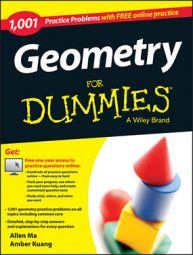In geometry, a transformation can change the size, location, or appearance of a geometric figure. Rigid motion refers to a limited transformation: only an object's location is changed, not its shape or size.

The following practice questions ask you to determine the rigid motion that will map one triangle onto another.

## Practice questions

1.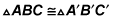What rigid motion would map one triangle onto the other?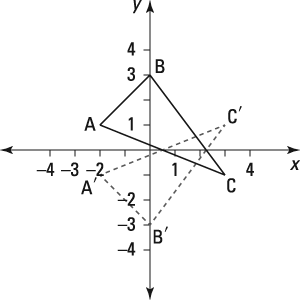2.What rigid motion would map one triangle onto the other?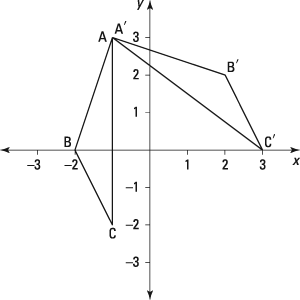1. rx–axis

The figure shows that the x-axis is the line of symmetry for the two triangles. When you reflect over the x-axis, you negate the sign of the original y value. In this figure, A (–2, 1) maps to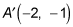Because the y value is negated, the point is reflected over the x-axis.

2. ry = –2x + 1

The figure shows that the line of symmetry has a y intercept at y = 1 and a slope of –2. Plugging this information into the slope-intercept form of a line makes y = –2x + 1 the line of symmetry for the two triangles.